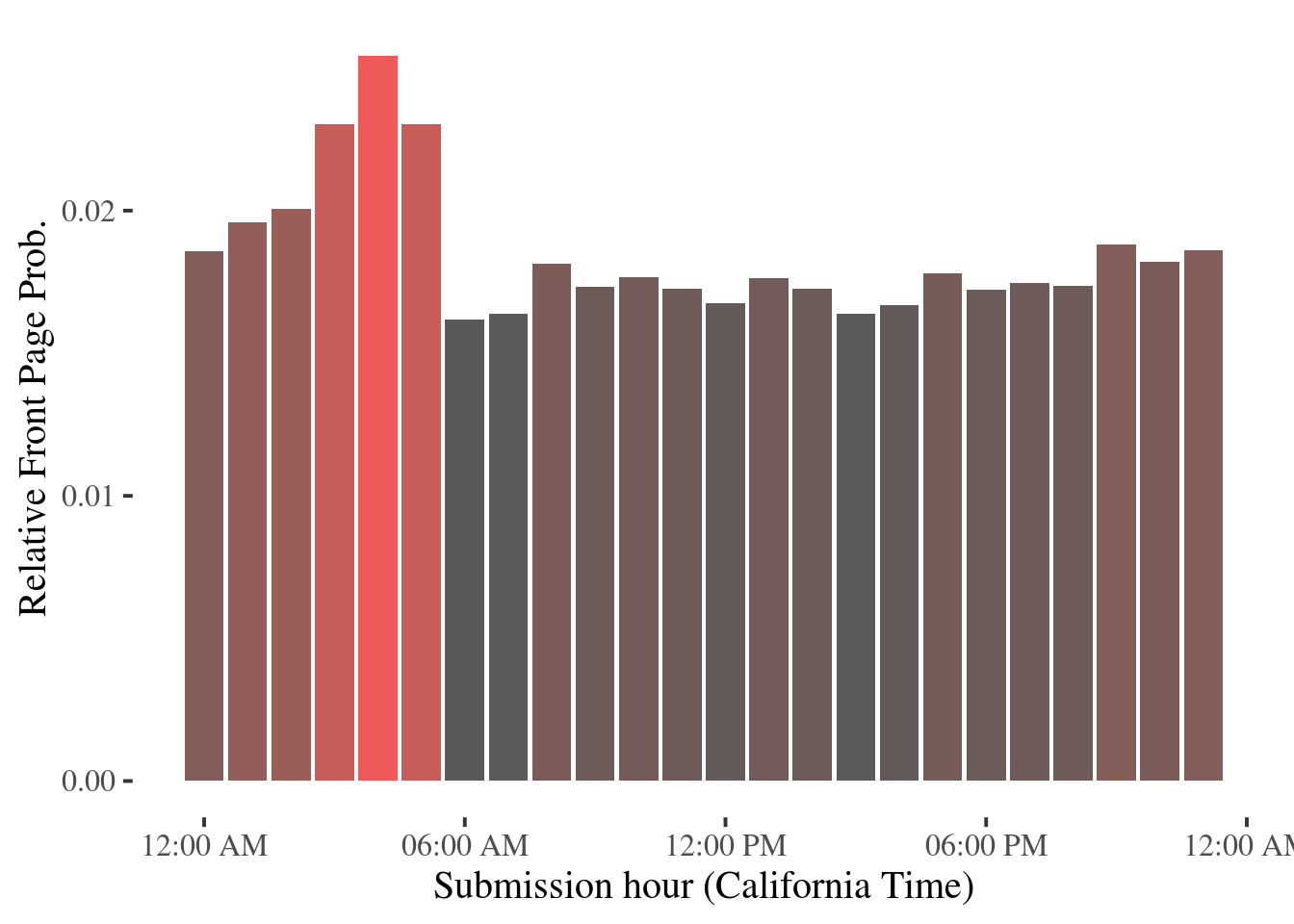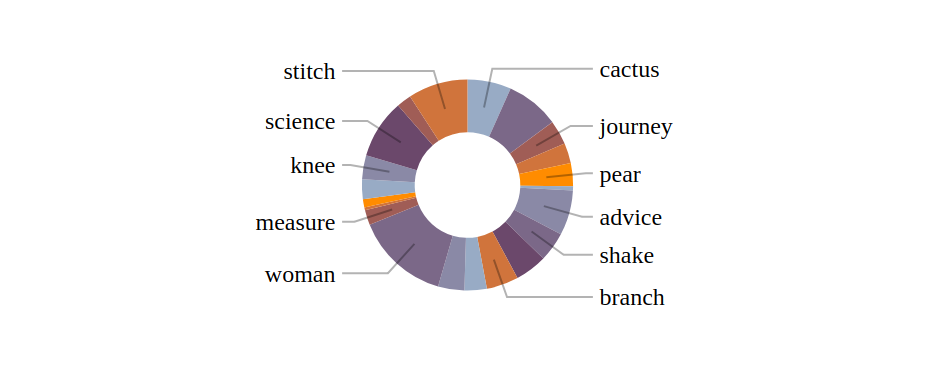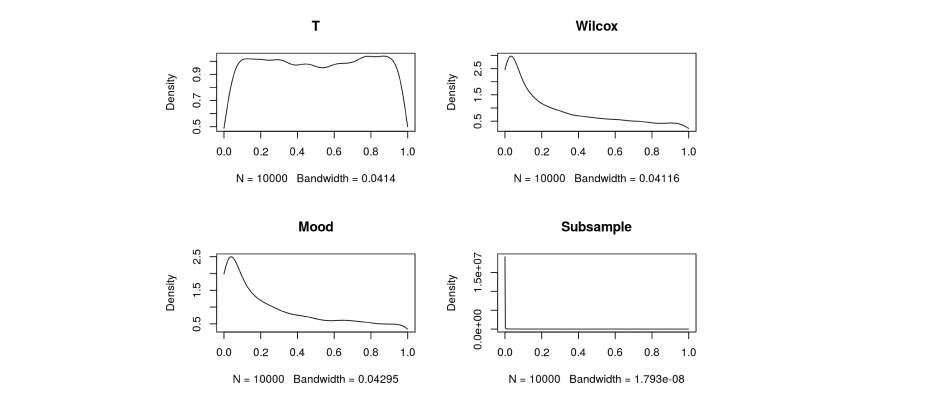# { [Idea]>>=stringify }

## Analyzing Hacker News Front Page Activities

Random and mildly interesting exploratory analysis and visualizations on Hack News activity data.## Pie Chart Callout Layout with Graph Algorithms

A small exercise on a simple graph algorithm, addressing the problem of dynamic pie chart label layout: given a set of vertically overlapping labels with some non-negative weights associated with them, return a subset, such that:

1. Labels in the subset do not overlap with each other
2. Maximizes sum of weights among all non-overlapping subsets## Anatomy of JavaScript Method Chaining

In this article, we will try to distill method chaining to its essence, making a distinction of mutable vs immutable chaining, and draw some parallels with composition in functional programming.

## Functional JavaScript: An Example of Equational Reasoning

In this article, I will demonstrate equational reasoning by focus on a key data structure in javaScript: Arrays. Alternatively, proving monad laws mathematically with javaScript syntax.

## Test Population Median Equality for Discrete Distributions

Simulation based examination of various statistical tests for median equality null for discrete r.v.: T-test, Wilcox rank sum, Mood Test and Bootstrapping. Findings:

• If we know nothing about the population, all of the proposed tests are not reliable

• Mood Test tends to reject more often, it might be a good test for contentious distributions, but turns out to be a poor fit for discrete distributions, which can have identical medians yet fail to satisfy Mood’s Test condition

• Subsampling/Bootstrapping tend to over-reject as well, especially when sample median is biased

• When population mean and population median can be assumed to be equal, T Test is very robust despite its obvious theoretical shortcomings

• Wilcox rank sum test is a general close second following T Test, and has good robustness